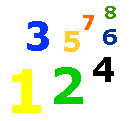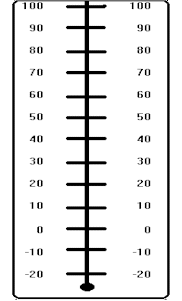# 1-1 How Hot or How Cold?

### Lesson List

 Look at the thermometer. Find 70 degrees.  70 degrees is just right for most people. Find 100 degrees.  100 degrees is a very, very hot day. Find 32 degrees.  When it is 32 degrees, water will freeze. Can you find 0 (zero) on the thermometer?  Do you see there are numbers below the 0?  In some places in the winter it gets very cold.  It goes below zero!Is 20 degrees above or below 30 degrees?

above
below

Is 32 degrees above or below 30 degrees?

above
below

Will it rain or snow if the temperature is 28 degrees?

rain
snow

Can you swim outdoors if the temperature is 90 degrees?

yes
no

Is -20 degrees above or below 0 (zero)?

above
below

The temperature in school is 70 degrees.  Is this a comfortable temperature for most people?

yes
no

Will an ice cream cone melt fast if the temperature is 90 degrees?

yes
no

Will a pond freeze if the temperature is 0 (zero)?

yes
no

Is 0 (zero) above or below -10 degrees?

above
below

Which temperature is hotter?

80 degrees
40 degrees

Which temperature is colder?

10 degrees
-10 degrees

Which temperature is colder than 0 (zero)?

32 degrees
-20 degrees